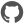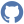# Contours

Namespace: XPlot.Plotly
Parent Module: Graph

### Constructors

 Constructor Description ``` new() ``` Signature: unit -> ContoursCompiledName: `.ctor`

### Instance members

 Instance member Description ``` coloring() ``` Signature: unit -> objDetermines the coloring method showing the contour values. If fill, coloring is done evenly between each contour level If heatmap, a heatmap gradient is coloring is applied between each contour level. If lines, coloring is done on the contour lines. If none, no coloring is applied on this trace. CompiledName: `set_coloring` ``` coloring() ``` Signature: unit -> unitDetermines the coloring method showing the contour values. If fill, coloring is done evenly between each contour level If heatmap, a heatmap gradient is coloring is applied between each contour level. If lines, coloring is done on the contour lines. If none, no coloring is applied on this trace. CompiledName: `get_coloring` ``` end() ``` Signature: unit -> floatSets the end contour level value. CompiledName: `set_end` ``` end() ``` Signature: unit -> unitSets the end contour level value. CompiledName: `get_end` ``` ShouldSerializecoloring() ``` Signature: unit -> bool``` ShouldSerializeend() ``` Signature: unit -> bool``` ShouldSerializeshowlines() ``` Signature: unit -> bool``` ShouldSerializesize() ``` Signature: unit -> bool``` ShouldSerializestart() ``` Signature: unit -> bool``` ShouldSerializex() ``` Signature: unit -> bool``` ShouldSerializey() ``` Signature: unit -> bool``` ShouldSerializez() ``` Signature: unit -> bool``` showlines() ``` Signature: unit -> boolDetermines whether or not the contour lines are drawn. Has only an effect if `contours.coloring` is set to fill. CompiledName: `set_showlines` ``` showlines() ``` Signature: unit -> unitDetermines whether or not the contour lines are drawn. Has only an effect if `contours.coloring` is set to fill. CompiledName: `get_showlines` ``` size() ``` Signature: unit -> floatSets the step between each contour level. CompiledName: `set_size` ``` size() ``` Signature: unit -> unitSets the step between each contour level. CompiledName: `get_size` ``` start() ``` Signature: unit -> floatSets the starting contour level value. CompiledName: `set_start` ``` start() ``` Signature: unit -> unitSets the starting contour level value. CompiledName: `get_start` ``` x() ``` Signature: unit -> XCompiledName: `set_x` ``` x() ``` Signature: unit -> unitCompiledName: `get_x` ``` y() ``` Signature: unit -> YCompiledName: `set_y` ``` y() ``` Signature: unit -> unitCompiledName: `get_y` ``` z() ``` Signature: unit -> ZCompiledName: `set_z` ``` z() ``` Signature: unit -> unitCompiledName: `get_z`